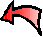##### Inches to Decimal Foot

This is a table of conversions from inches to decimal feet, useful for surveying.

Use the table or Calculator for either a foot with inches or a decimal of a foot and then click on "Convert" to get the equivalent measurement.

Inch 1 inch 0.0833 2 inches 0.167 3 inches 0.250 4 inches 0.333 5 inches 0.417 6 inches 0.500 7 inches 0.583 8 inches 0.667 9 inches 0.750 10 inches 0.833 11 inches 0.917 12 inches 1.000
1/8" is almost
1/100th
of a foot.
1" = 0.08
1 1/8" = 0.09
1 1/4" = 0.10
1 3/8" = 0.11
1 1/2" = 0.13
1 5/8" = 0.14
1 3/4" = 0.15
1 7/8" = 0.16
2" = 0.17

## Calculator

This calculates either way (feet/inches to decimal feet or vice versa).
Inches can have a fraction (3 1/4) or decimal (3.25 or 3,25).
FeetInches     Decimal Feet

Last Updated Sunday, November 13, 2011